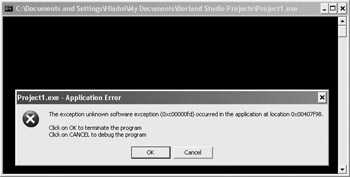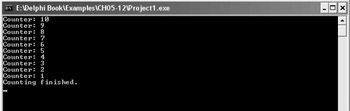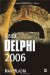Recursive Procedures and Functions

A recursive procedure is a procedure that can call itself. Actually, all procedures can call themselves, but if the call isn't properly implemented, the result is a bug (an infinite loop), not a recursive procedure. The following shows a poorly written recursive procedure:

procedure Bug; begin   Bug; end;

Calling the Bug procedure has disastrous results because the procedure will continue calling itself until it has used all available resources, and then it will crash (see Figure 5-7). If you run the application from the Delphi IDE, the Bug procedure will crash both the application and the entire IDE. The point is: Never write something that works like the Bug procedure.Figure 5-7: The result of infinite procedure recursion

A valid recursive procedure must have a way of controlling how many times it calls itself. The following procedure illustrates how you can use an integer parameter to implement a simple countdown. You can see the result of the CountDown procedure in Figure 5-8.Figure 5-8: The result of the CountDown procedure

Listing 5-12: A simple recursive procedureprogram Project1; {\$APPTYPE CONSOLE} uses   SysUtils; procedure CountDown(AStart: Integer); begin   WriteLn('Counter: ', AStart);   Dec(AStart);   if AStart = 0 then     WriteLn('Counting finished.')   else     CountDown(AStart); end; begin   CountDown(10);   ReadLn; end.The CountDown procedure recursively calls itself as long as the AStart parameter is greater than 0.

The most famous recursive function is the function that calculates factorials. The factorial is the product of all numbers in the range from 1 to n. For instance, the factorial of 4 is 24 (1 * 2 * 3 * 4).

Listing 5-13: A recursive functionprogram Project1; {\$APPTYPE CONSOLE} uses   SysUtils; function Factorial(ANumber: Integer): Integer; begin   if ANumber = 0 then     Result := 1   else     Result := ANumber * Factorial(ANumber - 1); end; begin   WriteLn('f(5) = ', Factorial(5)); { 120 }   ReadLn; end.Inside Delphi 2006 (Wordware Delphi Developers Library)
ISBN: 1598220039
EAN: 2147483647
Year: 2004
Pages: 212